Selina Solutions Concise Mathematics Class 6 Chapter 34 Mean and Median Exercise 34(A) help students understand the basic concepts, which are vital from the exam perspective. Finding the mean for a given set of numbers is the main concept discussed under this exercise. The solutions are designed in a step by step format to improve problem solving skills among students. Those who find Mathematics to be a difficult subject, are advised to follow these solutions on a daily basis. For a better hold on the concepts, students can refer to Selina Solutions Concise Mathematics Class 6 Chapter 34 Mean and Median Exercise 34(A) free PDF, from the links which are provided here.

## Selina Solutions Concise Mathematics Class 6 Chapter 34 Mean and Median Exercise 34(A) Download PDF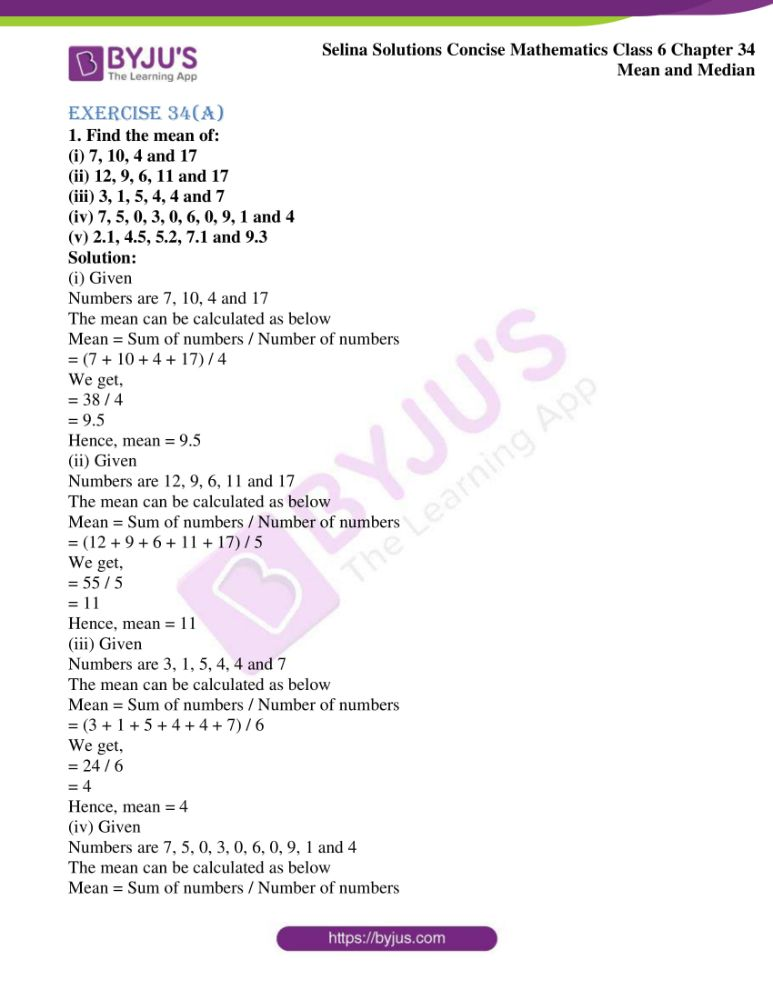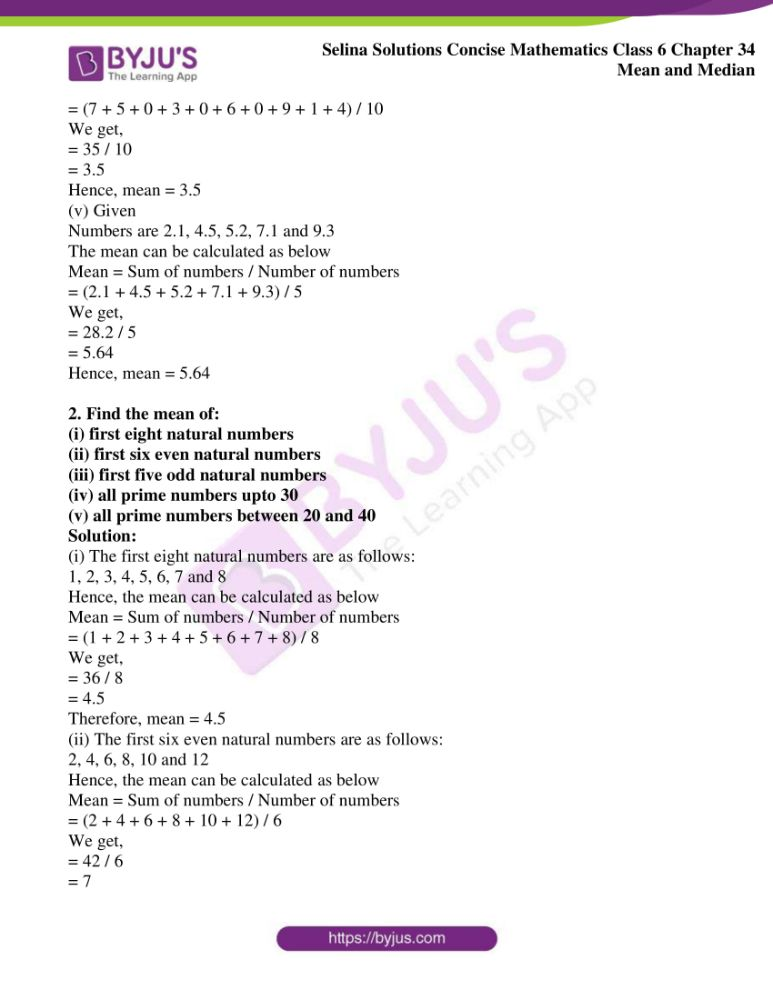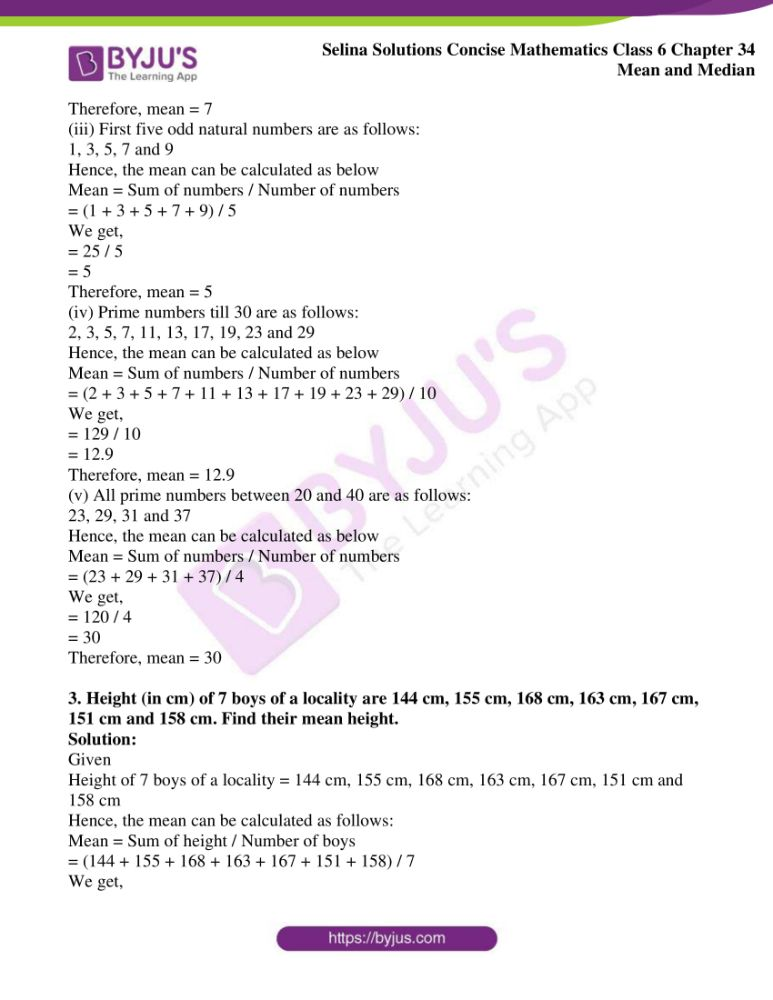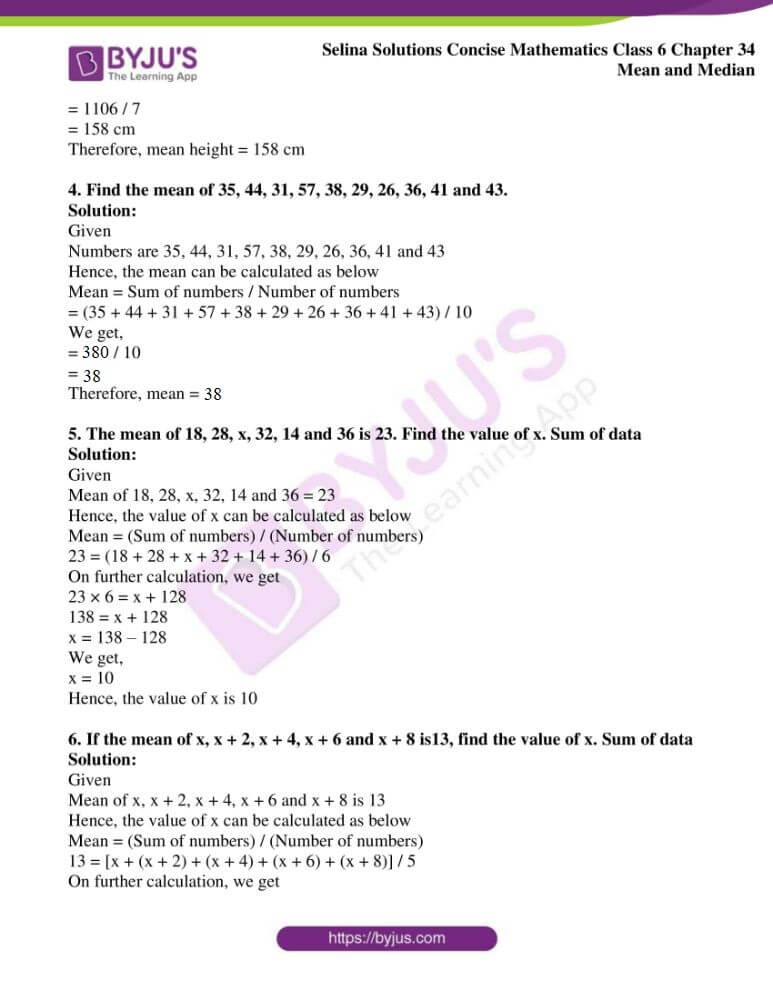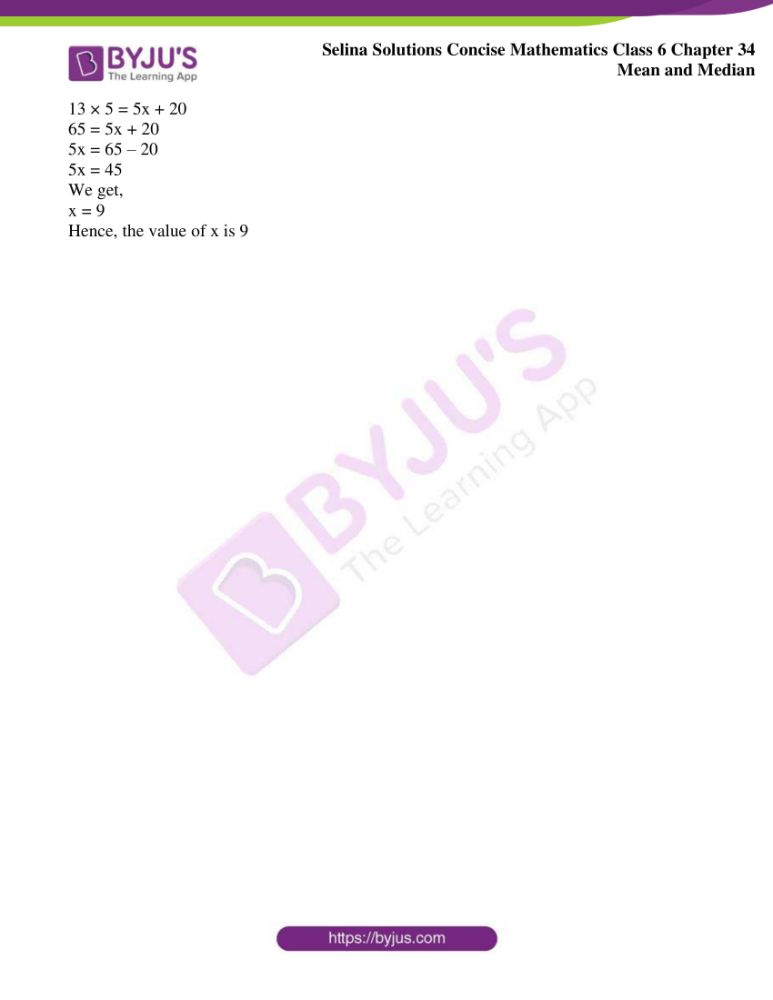### Access another exercise of Selina Solutions Concise Mathematics Class 6 Chapter 34 Mean and Median

Exercise 34(B) Solutions

### Access Selina Solutions Concise Mathematics Class 6 Chapter 34 Mean and Median Exercise 34(A)

Exercise 34(A)

1. Find the mean of:

(i) 7, 10, 4 and 17

(ii) 12, 9, 6, 11 and 17

(iii) 3, 1, 5, 4, 4 and 7

(iv) 7, 5, 0, 3, 0, 6, 0, 9, 1 and 4

(v) 2.1, 4.5, 5.2, 7.1 and 9.3

Solution:

(i) Given

Numbers are 7, 10, 4 and 17

The mean can be calculated as below

Mean = Sum of numbers / Number of numbers

= (7 + 10 + 4 + 17) / 4

We get,

= 38 / 4

= 9.5

Hence, mean = 9.5

(ii) Given

Numbers are 12, 9, 6, 11 and 17

The mean can be calculated as below

Mean = Sum of numbers / Number of numbers

= (12 + 9 + 6 + 11 + 17) / 5

We get,

= 55 / 5

= 11

Hence, mean = 11

(iii) Given

Numbers are 3, 1, 5, 4, 4 and 7

The mean can be calculated as below

Mean = Sum of numbers / Number of numbers

= (3 + 1 + 5 + 4 + 4 + 7) / 6

We get,

= 24 / 6

= 4

Hence, mean = 4

(iv) Given

Numbers are 7, 5, 0, 3, 0, 6, 0, 9, 1 and 4

The mean can be calculated as below

Mean = Sum of numbers / Number of numbers

= (7 + 5 + 0 + 3 + 0 + 6 + 0 + 9 + 1 + 4) / 10

We get,

= 35 / 10

= 3.5

Hence, mean = 3.5

(v) Given

Numbers are 2.1, 4.5, 5.2, 7.1 and 9.3

The mean can be calculated as below

Mean = Sum of numbers / Number of numbers

= (2.1 + 4.5 + 5.2 + 7.1 + 9.3) / 5

We get,

= 28.2 / 5

= 5.64

Hence, mean = 5.64

2. Find the mean of:

(i) first eight natural numbers

(ii) first six even natural numbers

(iii) first five odd natural numbers

(iv) all prime numbers upto 30

(v) all prime numbers between 20 and 40

Solution:

(i) The first eight natural numbers are as follows:

1, 2, 3, 4, 5, 6, 7 and 8

Hence, the mean can be calculated as below

Mean = Sum of numbers / Number of numbers

= (1 + 2 + 3 + 4 + 5 + 6 + 7 + 8) / 8

We get,

= 36 / 8

= 4.5

Therefore, mean = 4.5

(ii) The first six even natural numbers are as follows:

2, 4, 6, 8, 10 and 12

Hence, the mean can be calculated as below

Mean = Sum of numbers / Number of numbers

= (2 + 4 + 6 + 8 + 10 + 12) / 6

We get,

= 42 / 6

= 7

Therefore, mean = 7

(iii) First five odd natural numbers are as follows:

1, 3, 5, 7 and 9

Hence, the mean can be calculated as below

Mean = Sum of numbers / Number of numbers

= (1 + 3 + 5 + 7 + 9) / 5

We get,

= 25 / 5

= 5

Therefore, mean = 5

(iv) Prime numbers till 30 are as follows:

2, 3, 5, 7, 11, 13, 17, 19, 23 and 29

Hence, the mean can be calculated as below

Mean = Sum of numbers / Number of numbers

= (2 + 3 + 5 + 7 + 11 + 13 + 17 + 19 + 23 + 29) / 10

We get,

= 129 / 10

= 12.9

Therefore, mean = 12.9

(v) All prime numbers between 20 and 40 are as follows:

23, 29, 31 and 37

Hence, the mean can be calculated as below

Mean = Sum of numbers / Number of numbers

= (23 + 29 + 31 + 37) / 4

We get,

= 120 / 4

= 30

Therefore, mean = 30

3. Height (in cm) of 7 boys of a locality are 144 cm, 155 cm, 168 cm, 163 cm, 167 cm, 151 cm and 158 cm. Find their mean height.

Solution:

Given

Height of 7 boys of a locality = 144 cm, 155 cm, 168 cm, 163 cm, 167 cm, 151 cm and 158 cm

Hence, the mean can be calculated as follows:

Mean = Sum of height / Number of boys

= (144 + 155 + 168 + 163 + 167 + 151 + 158) / 7

We get,

= 1106 / 7

= 158 cm

Therefore, mean height = 158 cm

4. Find the mean of 35, 44, 31, 57, 38, 29, 26, 36, 41 and 43.

Solution:

Given

Numbers are 35, 44, 31, 57, 38, 29, 26, 36, 41 and 43

Hence, the mean can be calculated as below

Mean = Sum of numbers / Number of numbers

= (35 + 44 + 31 + 57 + 38 + 29 + 26 + 36 + 41 + 43) / 10

We get,

= 380 / 10

= 38

Therefore, mean = 38

5. The mean of 18, 28, x, 32, 14 and 36 is 23. Find the value of x. Sum of data

Solution:

Given

Mean of 18, 28, x, 32, 14 and 36 = 23

Hence, the value of x can be calculated as below

Mean = (Sum of numbers) / (Number of numbers)

23 = (18 + 28 + x + 32 + 14 + 36) / 6

On further calculation, we get

23 × 6 = x + 128

138 = x + 128

x = 138 – 128

We get,

x = 10

Hence, the value of x is 10

6. If the mean of x, x + 2, x + 4, x + 6 and x + 8 is13, find the value of x. Sum of data

Solution:

Given

Mean of x, x + 2, x + 4, x + 6 and x + 8 is 13

Hence, the value of x can be calculated as below

Mean = (Sum of numbers) / (Number of numbers)

13 = [x + (x + 2) + (x + 4) + (x + 6) + (x + 8)] / 5

On further calculation, we get

13 × 5 = 5x + 20

65 = 5x + 20

5x = 65 – 20

5x = 45

We get,

x = 9

Hence, the value of x is 9